# The convolution operation

This article covers the content discussed in The Convolutional Operation module of the Deep Learning course and all the images are taken from the same module.

So far, we have seen fully connected neural networks. In this article, we will discuss the convolution operation which is used in the Convolutional Neural Networks.

Before that, let’s see the key points of the Feed Forward Neural Network:

• Universal Approximation Theorem(UAT) says that Deep Neural Networks(DNN) are powerful function approximators.
• DNNs can be trained using backpropagation.
• However, Fully Connected DNNs(fully connected network means that any neuron in any of the layers is connected to all the neurons in the previous layer) are prone to overfitting as the network is very deep and the no. of parameters are very large which might result in the overfitting of the model.
• And the second problem with the fully connected networks is that some gradients might vanish due to long chains. Since the network is very deep, the gradients in the few of the starting layers might get vanished when flowing back and therefore resulting in no training of the weights.
• So, the objective is to have a network that is a complex network(having non-linearities) as we know that in most of the real-world problems the output is going to be a complex function of the input but has fewer parameters and is, therefore, less prone to overfitting. And CNN's belong to the family of networks that serves this objective.

Convolutional Operation means for a given input we re-estimate it as the weighted average of all the inputs around it. We have some weights assigned to the neighbor values and we take the weighted sum of the neighbor values to estimate the value of the current input/pixel.

For a 2D input, the classic input would be an image, where we re-calculate the value of every pixel by taking the weighted sum of pixels(neighbors) around it for example: let’s say the input image is as given below

Now in this input image, we calculate the value of each and every pixel by considering the weighted sum of pixels around it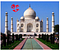Here we are calculating the value of circled pixel considering 3 neighbors around it, assume that the weights w1, w2, w3, w4 are associated with these 4 pixels respectively

Now, this matrix of weights is referred to as the Kernel or Filter. In the above case, we have the kernel of size 2X2.

We compute the output(re-estimated value of current pixel) using the following formula: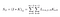Here m refers to the number of rows(which is 2 in this case) and n refers to the number of columns(which is 2 i this case).

Now we place the 2X2 filter over the first 2X2 portion of the image and take the weighted sum and that would give the new value of the first pixel.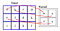We map the 2X2 kernel/filter over the 2X2 portion of the input.

The output of this operation would be: (aw + bx + ey + fz)

Then we move the filter horizontally by one and place it over the next 2 X 2 portion of the input; in this case pixels of interest would be b, c, f, g and we compute the output using the same technique and we would get:

And then again we move the kernel/filter by 1 in the horizontal direction and take the weighted sum.

So, after this, the output from the first layer would look like:

Then we move the kernel by 1 down in the vertical direction, calculate the output, move the kernel in the horizontal direction and in general we move the kernel like this: first, we start off with the starting portion of the image, move the filter in the horizontal direction and cover this row completely then we move the filter in the vertical direction(by some amount respective to top left portion of image), again stride it horizontally through the entire row and continue like this. In essence, we move the kernel left to right top to bottom.

Instead of considering pixels only in the forward direction, we consider previous neighbors as well

And to consider the previous neighbors, the formula for computing the output would be:

We take the limits from -m/2 to m/2 i.e we take half of the rows from previous neighbors and the other half from the forward direction(forward neighbors) and the same is the case in the vertical direction(-n/2 to n/2).

Typically, we take the odd-dimensional kernel.

Let the input image be as given below:

and we use kernel/filter of size 3X3 and for each pixel, we take the 3 X 3 neighborhood around it(pixel itself is a part of this 3 X 3 neighborhood and would be at the center) just like in the below image: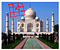Input Image, we consider 3X3 portions of this image as the kernel is of size 3X3

Let’s say this input is a 30X30 image, we go over every pixel systematically, place the filter such that the pixel is at the center of the kernel and re-estimate the value of that pixel as the weighted sum of pixels around it.

So, in this way, we get back the re-estimated value of all the pixels.

We all have seen the convolutional operation in practice. Let’s say the kernel that we are using is as below:

So, we move this kernel all over the image and re-compute every pixel as the weighted sum of the neighborhood. In this case, since all the weights are 1/9 that means the re-estimated value of each and every pixel would be 1/9th of its original value. This kernel is taking the average of all the 9 pixels over which this kernel would be placed.

That means for each pixel/color in the image, if we take the average(divide the weighted sum value by 9), it would dilute the value/blurs the image and the output we get by applying this convolutional operation is:

So, the blur operation that we all might have used in any of the photo editing application actually applies the convolution operation behind the scenes.

Now in the below-mentioned scenario, we are using 5 as the weight for the central pixel and 0 for the all the boundary pixels and -1 for the remaining pixels, so the net effect would be that the value/color intensity of the central pixel is boosted and its neighborhood information is getting subtracted so the result of this is that it sharpens the image.

The output of the above convolutional is:

Let’s take one more example: in the below case, the value for the central pixel is -8 and for all other pixels it is 1, so if we have the same color in the 3X3 portion of the image(just like for the marked pixel in the below image), let say the pixel intensity for this current pixel is denoted by ‘x’ then we get (8x from the central pixel and -8x from the weighted sum of all other pixels and summation of the these results into 0).

So, wherever we have the same color in the 3X3 portion(some sample regions marked in the below image) or to say the neighbors are exactly the same as the current pixel, we get the output intensity as 0.

So, in effect, what will happen is that where ever there is a boundary(yellow highlighted in the below image), there the neighboring pixels can not be the same as the current pixel, only in such regions we get the non-zero value, everywhere else we get a zero value. So, in effect, we end up detecting all the edges in the input image.

Below is a complete picture of how the 2D convolutional operation is performed over the input, we start with the top left corner, apply the kernel over that area, move the kernel horizontally towards right and once we have reached the end(completed the entire row) on the right side, we move the kernel downwards by some steps and again start from the left side and move towards right: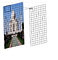We slide the kernel horizontally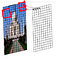Once we complete the entire row, we slide the kernel vertically in downwards direction and start from the left side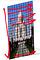We move from left to right and from top to bottom.

In the case of 3D input(image is also a 3D input as it has 3 channels corresponding to Red, Green, Blue, all these channels are superimposed on each other and that’s how we get the final image. In other words, every pixel in the image has 3 values associated with it, so we can look at that as the depth), we have 3 channels(depth) one corresponding to each of the RGB in the image, we use the filter of the same depth as the input and place the filter over the input and compute the weighted sum across all the 3 dimensions.

In most cases when we use convolution for 3D inputs, we use a 3D convolution filter(as depicted in the below image) that means if we place the filter at a given location in the image, we would take a weighted average of its 3D neighborhood but we are not going to slide it along the depth. What this conveys is that the kernel would have the same depth as the original input and that’s why there is no scope to move it through the depth/input. For example, the input image depth is 3 and the kernel depth is also 3 so there is no scope to move it along the depth. There is no movement available there

In this case, also, we move the filter horizontally and vertically as in the 2D case. We don’t move the filter along the depth as the input image depth is the same as the filter depth and there is no scope to move across the depth.

So, what we do in practice is we have this 3D kernel, we will start moving it, we will move it along the horizontal direction first, and we keep doing this through the entire image and once we reach the last box(we move from left to right and top to bottom), at the end of this, although our input was 3 dimensional, we get back a 2D output.

Points to consider:

• Input is 3D
• The filter is also 3D
• The convolutional operation that we perform is 2D as we are sliding the filter horizontally and vertically and not along the depth
• This is because the depth of the filter is the same as the depth of the input

In practice, we apply multiple kernels/filters to the same input and get the different representations/output from the same input as per the kernel used for example one filter might detect the vertical edges in the input, second might detect the horizontal edges in the image, another filter might blur the image and so on.

In the above image, we are using 3 different filters and we are getting 3 outputs corresponding to each filter. We can combine these different outputs representations into one single volume(each of the output representation would have width and height and after combining all of the representations we get the depth as well). So, if we apply 3 filters to the input, we get an output of depth 3, if we apply 100 filters to the input, we get the output of depth 100.

Points to consider:

• Each filter applied to a 3D input would give a 2D output.
• Combining the output of multiple such filters would result in a 3D output.

Let’s define some terminology and find out the relation between the input dimensions and the output dimensions:

The spatial extent(extent of the neighborhood we are looking at) of a filter(F) means the dimension of the filter, it would be ‘F X F’. Usually, we have an odd-dimensional filter and the depth of the filter would be the same as the depth of the input(Di in this case).

Now we want to relate the output dimensions with the input dimensions:

Let’s take 2D input of dimension ‘7 X 7’ and we have a filter of size ‘3 X 3’ over it.

As we slide the filter over it(from left to right and top to bottom), we keep computing the output values, and it's very clear that the output is smaller than the input.

This is how we slide the filter over the image:

The reason is obvious why this is happening, we can’t place the kernel at the corners as it will cross the boundary

We can’t place the filter at the crossed pixel(below image) because if we place it there then yellow highlighted portion would be undefined:

And in practice, we would stop at the crossed pixel(as in the below image) when the filter completely lies inside the image:

And this is why we get the smaller output because we would not be able to apply the filter in any part in the shaded region in the below image:

Hence for every pixel in the input, we are not computing the re-estimated value and therefore the number of pixels in the output is less than the number of pixels in the input.

This was the case for ‘3 X 3’ kernel, now let’s see what happens when we have ‘5 X 5’ kernel:

Now we can not place the kernel at the crossed pixel in the above image. We can not place the kernel at the yellow highlighted pixel as well. So, in this case, we can not place the kernel at any of the shaded regions in the below image:

The bigger the kernel used, the smaller is the output.

So, the output dimension in terms of the input is:

If we want the output to be the same size as the input, then we need to pad the input appropriately: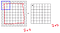Here we pad the input with 0 all over the input image and apply the 3X3 filter over the input and we get the output of the same dimension as the input

If we place the kernel at the crossed pixel in the below image, we now have 5 artificial pixels with a value of 0 and we would be able to re-estimate the value of this crossed pixel.

Now the output would be again ‘7 X 7’ as we have introduced this artificial boundary around the original input and this boundary contains all the values as 0.

If we have a ‘5 X 5’ filter, it would still go outside the image even after this artificial padding

So, in this case, we need to increase padding. Earlier we added padding of 1(meaning 1 row at the top, 1 at the bottom, 1 at the left and 1 at the right). And it’s obvious from the above image that if we want to use a ‘5 X 5’, then we should use the padding of 2.

The bigger the kernel size the larger is the padding required and the updated formula for the relation between input and output dimension is:

Stride(S): Stride defines the interval at which the filter is applied, till now we discussed all the cases considering stride to be 1 as we’re moving the filter by 1 in the horizontal and vertical direction as depicted in the below image:

In some cases, we may not want this to say we don’t want a full replica of the image and just need a summary of it. In that case, we may choose to apply the filter only at alternate locations in the input.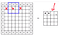Here we use S = 2 i.e we move the filter by 2 in the horizontal as well as the vertical direction

This interval between two successive pixels where we apply the kernel is termed as the Stride. And in the above case, the output would be roughly half the input as we are skipping part of the image by 1 every time.

Now, if we are using a stride ‘S’, then the formula to compute the width and height is given by:

Higher the stride, the smaller is the size of the output.

The depth of the output is going to be the same as the number of filters that we have.

Each 3D filter applied over 3D input would give one 2D output if we use K such filters, we get K such 2D outputs and if we stack up all these K outputs we get the depth of the output as K. So, the depth of the output is the same as the no. of filters used.